# Quick Reference

Temperature - °C or °K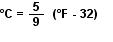Pressure SI unit – Pascal
1 Pa = 1 N/m2, too small a unit
Common unit = Bar
1 Bar =105 N/m2= 0.1 Mpa
Atmospheric Pressure = 1 Bar abs at MSL
Vacuum = 0 Bar abs

Gauge pressure + Atmospheric pressure = Absolute pressure

barg = kg/cm2g

Density in kg/m3
Specific Volume = 1 / Density in m
3/kg
Specific Gravity = Density ratio to water

Energy SI unit = 1 Joule = 1 Nm = 4.186 cal
Common unit = kilocalorie
1 kcal = heat reqd to raise 1 kg water by 1°C
1 kcal = 4186.8 Joules
Cp = sp. heat capacity in kcal/kg °C

Conversions between SI and other units

mWC = meters water column
1 Bar = 10 mWC

1 Bar = 14.23 PSI (Lbs/in2)
150 psi = 10.54 Kg/cm2g
50 psi = 3.5 Kg/cm2g

10 bar g = 11 bar a = 10.2 kg/cm2g = 11.2 kg/cm2a = 145 psig = 1 MPa = 106 N/m2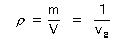Where
ρ= Density(kg/m
3)
m = Mass (kg)
V= Volume (m
3)
vg= Specific volume (m
3/kg)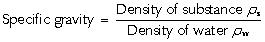Enthalpy of saturated steam

hg = hf + hfg

Where:
hg = Total enthalpy or total heat of saturated steam (kJ/kg)
hf = Liquid enthalpy (Sensible heat) (kJ/kg)
hfg = Enthalpy of evaporation (Latent heat) (kJ/kg)

Heat Balance in Process

Primary Q = m x hfg

Where,
Primary Q = Quantity of heat energy released (in kcals)
m = Mass of steam releasing the heat (in kgs)
hfg = Specific enthalpy of evaporation of steam (in kcals/kg)

Secondary Q = m x cp x ΔT

Where,
Secondary Q = Quantity of heat energy absorbed (in kcals)
m = Mass of the substance absorbing the heat (in kgs)
cp = Specific heat capacity of the substance (in kcals / kg °C )
ΔT = Temperature rise of the substance (in °C)

Primary Q = Secondary Q

Heat transfer equation:

Where:
Q = Heat transferred per unit time (kcals/hr)
U = Overall heat transfer coefficient (kcals/hr / m²°C)
A = Heat transfer area (m²)
ΔT = Temperature difference between the primary and secondary fluid (°C)

Steam line sizing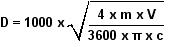Where,
D = Line size in mm
m = Mass flowrate of steam in kg/h
V = Specific volume in m3/kg
π = a constant 3.14
c = velocity m/s

Calculating Savings:

Boiler Heat Input = Qf x GCV

where,
Qf = Quantity of fuel (in kg/hr)
GCV (Gross Calorific Value) =Energy contained in fuel in kcal/kg

Boiler Heat Output = Qs x (Hs – Hw)

Where,
Qs = Quantity of steam (in kg/hr)
Hs = Heat contained in steam (Enthalpy of Saturated steam hg)
Hw = Heat already present in the water from which steam is raised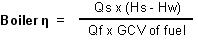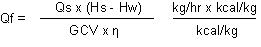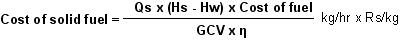where,
Qc = Quantity of condensate (in kg/hr)
Hc = Heat contained in condensate (in kcal/kg)
Hw = Heat already present in the water at ambient temperature (in kcal/kg)
GCV (Gross Calorific Value) =Energy contained in fuel in kcal/kg
η = Boiler efficiency
ρ = Specific gravity of liquid/gas fuel

% Flash steam calculation: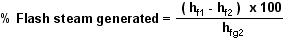Where,
hf1 = Enthalpy of water at the higher pressure kcal/kg
hf2 = Enthalpy of water at the flashing pressure kcal/kg
hfg2 = Enthalpy of evaporation at the flash steam pressure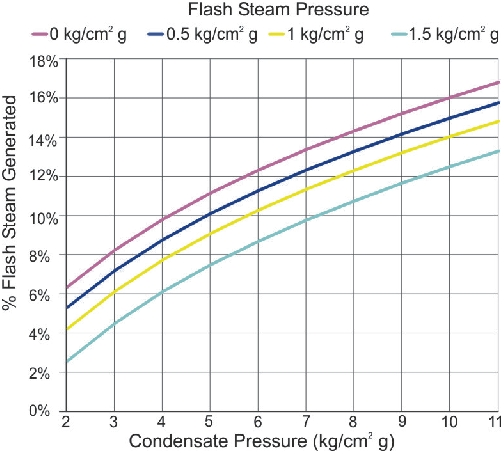Actual rating of a Boiler

Actual Rating = F&A Rating X 540 / ( hg – hfFW)

where,
hg = enthalpy of steam at generation pressure
hfFW = Feed water enthalpy

Blowdown: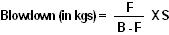Where,
F = Feedwater TDS in ppm
B = Boiler water set point in ppm
S = Steam generation in kg/hr.